Chemistry

# 14.3Relative Strengths of Acids and Bases

Chemistry14.3 Relative Strengths of Acids and Bases

### Learning Objectives

By the end of this section, you will be able to:
• Assess the relative strengths of acids and bases according to their ionization constants
• Rationalize trends in acid–base strength in relation to molecular structure
• Carry out equilibrium calculations for weak acid–base systems

We can rank the strengths of acids by the extent to which they ionize in aqueous solution. The reaction of an acid with water is given by the general expression:

$HA(aq)+H2O(l)⇌H3O+(aq)+A−(aq)HA(aq)+H2O(l)⇌H3O+(aq)+A−(aq)$
14.40

Water is the base that reacts with the acid HA, A is the conjugate base of the acid HA, and the hydronium ion is the conjugate acid of water. A strong acid yields 100% (or very nearly so) of $H3O+H3O+$ and A when the acid ionizes in water; Figure 14.6 lists several strong acids. A weak acid gives small amounts of $H3O+H3O+$ and A.

Figure 14.6 Some of the common strong acids and bases are listed here.

The relative strengths of acids may be determined by measuring their equilibrium constants in aqueous solutions. In solutions of the same concentration, stronger acids ionize to a greater extent, and so yield higher concentrations of hydronium ions than do weaker acids. The equilibrium constant for an acid is called the acid-ionization constant, Ka. For the reaction of an acid HA:

$HA(aq)+H2O(l)⇌H3O+(aq)+A−(aq),HA(aq)+H2O(l)⇌H3O+(aq)+A−(aq),$
14.41

we write the equation for the ionization constant as:

$Ka=[H3O+][A−][HA]Ka=[H3O+][A−][HA]$
14.42

where the concentrations are those at equilibrium. Although water is a reactant in the reaction, it is the solvent as well, so we do not include [H2O] in the equation. The larger the Ka of an acid, the larger the concentration of $H3O+H3O+$ and A relative to the concentration of the nonionized acid, HA. Thus a stronger acid has a larger ionization constant than does a weaker acid. The ionization constants increase as the strengths of the acids increase. (A table of ionization constants of weak acids appears in Appendix H, with a partial listing in Table 14.2.)

The following data on acid-ionization constants indicate the order of acid strength CH3CO2H < HNO2 < $HSO4−:HSO4−:$

$CH3CO2H(aq)+H2O(l)⇌H3O+(aq)+CH3CO2−(aq)Ka=1.8×10−5CH3CO2H(aq)+H2O(l)⇌H3O+(aq)+CH3CO2−(aq)Ka=1.8×10−5$
14.43
$HNO2(aq)+H2O(l)⇌H3O+(aq)+NO2−(aq)Ka=4.6×10−4HNO2(aq)+H2O(l)⇌H3O+(aq)+NO2−(aq)Ka=4.6×10−4$
14.44
$HSO4−(aq)+H2O(aq)⇌H3O+(aq)+SO42−(aq)Ka=1.2×10−2HSO4−(aq)+H2O(aq)⇌H3O+(aq)+SO42−(aq)Ka=1.2×10−2$
14.45

Another measure of the strength of an acid is its percent ionization. The percent ionization of a weak acid is the ratio of the concentration of the ionized acid to the initial acid concentration, times 100:

$% ionization=[H3O+]eq[HA]0×100% ionization=[H3O+]eq[HA]0×100$
14.46

Because the ratio includes the initial concentration, the percent ionization for a solution of a given weak acid varies depending on the original concentration of the acid, and actually decreases with increasing acid concentration.

### Example 14.7

#### Calculation of Percent Ionization from pH

Calculate the percent ionization of a 0.125-M solution of nitrous acid (a weak acid), with a pH of 2.09.

#### Solution

The percent ionization for an acid is:
$[H3O+]eq[HNO2]0×100[H3O+]eq[HNO2]0×100$
14.47

The chemical equation for the dissociation of the nitrous acid is: $HNO2(aq)+H2O(l)⇌NO2−(aq)+H3O+(aq).HNO2(aq)+H2O(l)⇌NO2−(aq)+H3O+(aq).$ Since 10−pH = $[H3O+],[H3O+],$ we find that 10−2.09 = 8.1 $××$ 10−3 M, so that percent ionization is:

$8.1×10−30.125×100=6.5%8.1×10−30.125×100=6.5%$
14.48

Remember, the logarithm 2.09 indicates a hydronium ion concentration with only two significant figures.

Calculate the percent ionization of a 0.10-M solution of acetic acid with a pH of 2.89.

1.3% ionized

We can rank the strengths of bases by their tendency to form hydroxide ions in aqueous solution. The reaction of a Brønsted-Lowry base with water is given by:

$B(aq)+H2O(l)⇌HB+(aq)+OH−(aq)B(aq)+H2O(l)⇌HB+(aq)+OH−(aq)$
14.49

Water is the acid that reacts with the base, HB+ is the conjugate acid of the base B, and the hydroxide ion is the conjugate base of water. A strong base yields 100% (or very nearly so) of OH and HB+ when it reacts with water; Figure 14.6 lists several strong bases. A weak base yields a small proportion of hydroxide ions. Soluble ionic hydroxides such as NaOH are considered strong bases because they dissociate completely when dissolved in water.

As we did with acids, we can measure the relative strengths of bases by measuring their base-ionization constant (Kb) in aqueous solutions. In solutions of the same concentration, stronger bases ionize to a greater extent, and so yield higher hydroxide ion concentrations than do weaker bases. A stronger base has a larger ionization constant than does a weaker base. For the reaction of a base, B:

$B(aq)+H2O(l)⇌HB+(aq)+OH−(aq),B(aq)+H2O(l)⇌HB+(aq)+OH−(aq),$
14.50

we write the equation for the ionization constant as:

$Kb=[HB+][OH−][B]Kb=[HB+][OH−][B]$
14.51

where the concentrations are those at equilibrium. Again, we do not include [H2O] in the equation because water is the solvent. The chemical reactions and ionization constants of the three bases shown are:

$NO2−(aq)+H2O(l)⇌HNO2(aq)+OH−(aq)Kb=2.22×10−11NO2−(aq)+H2O(l)⇌HNO2(aq)+OH−(aq)Kb=2.22×10−11$
14.52
$CH3CO2−(aq)+H2O(l)⇌CH3CO2H(aq)+OH−(aq)Kb=5.6×10−10CH3CO2−(aq)+H2O(l)⇌CH3CO2H(aq)+OH−(aq)Kb=5.6×10−10$
14.53
$NH3(aq)+H2O(l)⇌NH4+(aq)+OH−(aq)Kb=1.8×10−5NH3(aq)+H2O(l)⇌NH4+(aq)+OH−(aq)Kb=1.8×10−5$
14.54

A table of ionization constants of weak bases appears in Appendix I (with a partial list in Table 14.3). As with acids, percent ionization can be measured for basic solutions, but will vary depending on the base ionization constant and the initial concentration of the solution.

Consider the ionization reactions for a conjugate acid-base pair, HA − A:

$HA(aq)+H2O(l)⇌H3O+(aq)+A−(aq)Ka=[H3O+][A−][HA]HA(aq)+H2O(l)⇌H3O+(aq)+A−(aq)Ka=[H3O+][A−][HA]$
14.55
$A−(aq)+H2O(l)⇌OH−(aq)+HA(aq)Kb=[HA][OH][A−]A−(aq)+H2O(l)⇌OH−(aq)+HA(aq)Kb=[HA][OH][A−]$
14.56

Adding these two chemical equations yields the equation for the autoionization for water:

$HA(aq)+H2O(l)+A−(aq)+H2O(l)⇌H3O+(aq)+A−(aq)+OH−(aq)+HA(aq)HA(aq)+H2O(l)+A−(aq)+H2O(l)⇌H3O+(aq)+A−(aq)+OH−(aq)+HA(aq)$
14.57
$2H2O(l)⇌H3O+(aq)+OH−(aq)2H2O(l)⇌H3O+(aq)+OH−(aq)$
14.58

Because of the way mass-action expressions are defined, the K expression for any chemical equation that is derived by adding together two or more other equations is equal to the mathematical product of the other equations’ K expressions. Multiplying the mass-action expressions together and canceling common terms, we see that:

$Ka×Kb=[H3O+][A−][HA]×[HA][OH−][A−]=[H3O+][OH−]=KwKa×Kb=[H3O+][A−][HA]×[HA][OH−][A−]=[H3O+][OH−]=Kw$
14.59

For example, the acid ionization constant of acetic acid (CH3COOH) is 1.8 $××$ 10−5, and the base ionization constant of its conjugate base, acetate ion $(CH3COO−),(CH3COO−),$ is 5.6 $××$ 10−10. The product of these two constants is indeed equal to Kw:

$Ka×Kb=(1.8×10−5)×(5.6×10−10)=1.0×10−14=KwKa×Kb=(1.8×10−5)×(5.6×10−10)=1.0×10−14=Kw$
14.60

The extent to which an acid, HA, donates protons to water molecules depends on the strength of the conjugate base, A, of the acid. If A is a strong base, any protons that are donated to water molecules are recaptured by A. Thus there is relatively little A and $H3O+H3O+$ in solution, and the acid, HA, is weak. If A is a weak base, water binds the protons more strongly, and the solution contains primarily A and H3O+—the acid is strong. Strong acids form very weak conjugate bases, and weak acids form stronger conjugate bases (Figure 14.7).

Figure 14.7 This diagram shows the relative strengths of conjugate acid-base pairs, as indicated by their ionization constants in aqueous solution.

Figure 14.8 lists a series of acids and bases in order of the decreasing strengths of the acids and the corresponding increasing strengths of the bases. The acid and base in a given row are conjugate to each other.

Figure 14.8 The chart shows the relative strengths of conjugate acid-base pairs.

The first six acids in Figure 14.8 are the most common strong acids. These acids are completely dissociated in aqueous solution. The conjugate bases of these acids are weaker bases than water. When one of these acids dissolves in water, their protons are completely transferred to water, the stronger base.

Those acids that lie between the hydronium ion and water in Figure 14.8 form conjugate bases that can compete with water for possession of a proton. Both hydronium ions and nonionized acid molecules are present in equilibrium in a solution of one of these acids. Compounds that are weaker acids than water (those found below water in the column of acids) in Figure 14.8 exhibit no observable acidic behavior when dissolved in water. Their conjugate bases are stronger than the hydroxide ion; if any conjugate base were formed, it would react with water to form the hydroxide ion.

The extent to which a base forms hydroxide ion in aqueous solution depends on the strength of the base relative to that of the hydroxide ion, as shown in the last column in Figure 14.8. A strong base, such as one of those lying below hydroxide ion, accepts protons from water to yield 100% of the conjugate acid and hydroxide ion. Those bases lying between water and hydroxide ion accept protons from water, but a mixture of the hydroxide ion and the base results. Bases that are weaker than water (those that lie above water in the column of bases) show no observable basic behavior in aqueous solution.

### Example 14.8

#### The Product Ka$××$Kb = Kw

Use the Kb for the nitrite ion, $NO2−,NO2−,$ to calculate the Ka for its conjugate acid.

#### Solution

Kb for $NO2−NO2−$ is given in this section as 2.17 $××$ 10−11. The conjugate acid of $NO2−NO2−$ is HNO2; Ka for HNO2 can be calculated using the relationship:
$Ka×Kb=1.0×10−14=KwKa×Kb=1.0×10−14=Kw$
14.61

Solving for Ka, we get:

$Ka=KwKb=1.0×10−142.17×10−11=4.6×10−4Ka=KwKb=1.0×10−142.17×10−11=4.6×10−4$
14.62

This answer can be verified by finding the Ka for HNO2 in Appendix H.

We can determine the relative acid strengths of $NH4+NH4+$ and HCN by comparing their ionization constants. The ionization constant of HCN is given in Appendix H as 4.9 $××$ 10−10. The ionization constant of $NH4+NH4+$ is not listed, but the ionization constant of its conjugate base, NH3, is listed as 1.8 $××$ 10−5. Determine the ionization constant of $NH4+,NH4+,$ and decide which is the stronger acid, HCN or $NH4+.NH4+.$

$NH4+NH4+$ is the slightly stronger acid (Ka for $NH4+NH4+$ = 5.6 $××$ 10−10).

### The Ionization of Weak Acids and Weak Bases

Many acids and bases are weak; that is, they do not ionize fully in aqueous solution. A solution of a weak acid in water is a mixture of the nonionized acid, hydronium ion, and the conjugate base of the acid, with the nonionized acid present in the greatest concentration. Thus, a weak acid increases the hydronium ion concentration in an aqueous solution (but not as much as the same amount of a strong acid).

Acetic acid, CH3CO2H, is a weak acid. When we add acetic acid to water, it ionizes to a small extent according to the equation:

$CH3CO2H(aq)+H2O(l)⇌H3O+(aq)+CH3CO2−(aq),CH3CO2H(aq)+H2O(l)⇌H3O+(aq)+CH3CO2−(aq),$
14.63

giving an equilibrium mixture with most of the acid present in the nonionized (molecular) form. This equilibrium, like other equilibria, is dynamic; acetic acid molecules donate hydrogen ions to water molecules and form hydronium ions and acetate ions at the same rate that hydronium ions donate hydrogen ions to acetate ions to reform acetic acid molecules and water molecules. We can tell by measuring the pH of an aqueous solution of known concentration that only a fraction of the weak acid is ionized at any moment (Figure 14.9). The remaining weak acid is present in the nonionized form.

For acetic acid, at equilibrium:

$Ka=[H3O+][CH3CO2−][CH3CO2H]=1.8×10−5Ka=[H3O+][CH3CO2−][CH3CO2H]=1.8×10−5$
14.64
Figure 14.9 pH paper indicates that a 0.l-M solution of HCl (beaker on left) has a pH of 1. The acid is fully ionized and $[H3O+][H3O+]$ = 0.1 M. A 0.1-M solution of CH3CO2H (beaker on right) is a pH of 3 ($[H3O+][H3O+]$ = 0.001 M) because the weak acid CH3CO2H is only partially ionized. In this solution, $[H3O+][H3O+]$ < [CH3CO2H]. (credit: modification of work by Sahar Atwa)
Ionization Constants of Some Weak Acids
Ionization Reaction Ka at 25 °C
$HSO4−+H2O⇌H3O++SO42−HSO4−+H2O⇌H3O++SO42−$ 1.2 $××$ 10−2
$HF+H2O⇌H3O++F−HF+H2O⇌H3O++F−$ 3.5 $××$ 10−4
$HNO2+H2O⇌H3O++NO2−HNO2+H2O⇌H3O++NO2−$ 4.6 $××$ 10−4
$HCNO+H2O⇌H3O++NCO−HCNO+H2O⇌H3O++NCO−$ 2 $××$ 10−4
$HCO2H+H2O⇌H3O++HCO2−HCO2H+H2O⇌H3O++HCO2−$ 1.8 $××$ 10−4
$CH3CO2H+H2O⇌H3O++CH3CO2−CH3CO2H+H2O⇌H3O++CH3CO2−$ 1.8 $××$ 10−5
$HCIO+H2O⇌H3O++CIO−HCIO+H2O⇌H3O++CIO−$ 2.9 $××$ 10−8
$HBrO+H2O⇌H3O++BrO−HBrO+H2O⇌H3O++BrO−$ 2.8 $××$ 10−9
$HCN+H2O⇌H3O++CN−HCN+H2O⇌H3O++CN−$ 4.9 $××$ 10−10
Table 14.2

Table 14.2 gives the ionization constants for several weak acids; additional ionization constants can be found in Appendix H.

At equilibrium, a solution of a weak base in water is a mixture of the nonionized base, the conjugate acid of the weak base, and hydroxide ion with the nonionized base present in the greatest concentration. Thus, a weak base increases the hydroxide ion concentration in an aqueous solution (but not as much as the same amount of a strong base).

For example, a solution of the weak base trimethylamine, (CH3)3N, in water reacts according to the equation:

$(CH3)3N(aq)+H2O(l)⇌(CH3)3NH+(aq)+OH−(aq),(CH3)3N(aq)+H2O(l)⇌(CH3)3NH+(aq)+OH−(aq),$
14.65

giving an equilibrium mixture with most of the base present as the nonionized amine. This equilibrium is analogous to that described for weak acids.

We can confirm by measuring the pH of an aqueous solution of a weak base of known concentration that only a fraction of the base reacts with water (Figure 14.10). The remaining weak base is present as the unreacted form. The equilibrium constant for the ionization of a weak base, Kb, is called the ionization constant of the weak base, and is equal to the reaction quotient when the reaction is at equilibrium. For trimethylamine, at equilibrium:

$Kb=[(CH3)3NH+][OH−][(CH3)3N]Kb=[(CH3)3NH+][OH−][(CH3)3N]$
14.66
Figure 14.10 pH paper indicates that a 0.1-M solution of NH3 (left) is weakly basic. The solution has a pOH of 3 ([OH] = 0.001 M) because the weak base NH3 only partially reacts with water. A 0.1-M solution of NaOH (right) has a pOH of 1 because NaOH is a strong base. (credit: modification of work by Sahar Atwa)

The ionization constants of several weak bases are given in Table 14.3 and in Appendix I.

Ionization Constants of Some Weak Bases
Ionization Reaction Kb at 25 °C
$(CH3)2NH+H2O⇌(CH3)2NH2++OH−(CH3)2NH+H2O⇌(CH3)2NH2++OH−$ 5.9 $××$ 10−4
$CH3NH2+H2O⇌CH3NH3++OH−CH3NH2+H2O⇌CH3NH3++OH−$ 4.4 $××$ 10−4
$(CH3)3N+H2O⇌(CH3)3NH++OH−(CH3)3N+H2O⇌(CH3)3NH++OH−$ 6.3 $××$ 10−5
$NH3+H2O⇌NH4++OH−NH3+H2O⇌NH4++OH−$ 1.8 $××$ 10−5
$C6H5NH2+H2O⇌C6N5NH3++OH−C6H5NH2+H2O⇌C6N5NH3++OH−$ 4.3 $××$ 10−10
Table 14.3

### Example 14.9

#### Determination of Ka from Equilibrium Concentrations

Acetic acid is the principal ingredient in vinegar (Figure 14.11); that's why it tastes sour. At equilibrium, a solution contains [CH3CO2H] = 0.0787 M and $[H3O+]=[CH3CO2−]=0.00118M.[H3O+]=[CH3CO2−]=0.00118M.$ What is the value of Ka for acetic acid?
Figure 14.11 Vinegar is a solution of acetic acid, a weak acid. (credit: modification of work by “HomeSpot HQ”/Flickr)

#### Solution

We are asked to calculate an equilibrium constant from equilibrium concentrations. At equilibrium, the value of the equilibrium constant is equal to the reaction quotient for the reaction:
$CH3CO2H(aq)+H2O(l)⇌H3O+(aq)+CH3CO2−(aq)CH3CO2H(aq)+H2O(l)⇌H3O+(aq)+CH3CO2−(aq)$
14.67
$Ka=[H3O+][CH3CO2−][CH3CO2H]=(0.00118)(0.00118)0.0787=1.77×10−5Ka=[H3O+][CH3CO2−][CH3CO2H]=(0.00118)(0.00118)0.0787=1.77×10−5$
14.68

What is the equilibrium constant for the ionization of the $HSO4−HSO4−$ ion, the weak acid used in some household cleansers:
$HSO4−(aq)+H2O(l)⇌H3O+(aq)+SO42−(aq)HSO4−(aq)+H2O(l)⇌H3O+(aq)+SO42−(aq)$
14.69

In one mixture of NaHSO4 and Na2SO4 at equilibrium, $[H3O+][H3O+]$ = 0.027 M; $[HSO4−]=0.29M;[HSO4−]=0.29M;$ and $[SO42−]=0.13M.[SO42−]=0.13M.$

Ka for $HSO4−HSO4−$ = 1.2 $××$ 10−2

### Example 14.10

#### Determination of Kb from Equilibrium Concentrations

Caffeine, C8H10N4O2 is a weak base. What is the value of Kb for caffeine if a solution at equilibrium has [C8H10N4O2] = 0.050 M, $[C8H10N4O2H+][C8H10N4O2H+]$ = 5.0 $××$ 10−3 M, and [OH] = 2.5 $××$ 10−3 M?

#### Solution

At equilibrium, the value of the equilibrium constant is equal to the reaction quotient for the reaction:
$C8H10N4O2(aq)+H2O(l)⇌C8H10N4O2H+(aq)+OH−(aq)C8H10N4O2(aq)+H2O(l)⇌C8H10N4O2H+(aq)+OH−(aq)$
14.70
$Kb=[C8H10N4O2H+][OH−][C8H10N4O2]=(5.0×10−3)(2.5×10−3)0.050=2.5×10−4Kb=[C8H10N4O2H+][OH−][C8H10N4O2]=(5.0×10−3)(2.5×10−3)0.050=2.5×10−4$
14.71

What is the equilibrium constant for the ionization of the $HPO42−HPO42−$ ion, a weak base:
$HPO42−(aq)+H2O(l)⇌H2PO4−(aq)+OH−(aq)HPO42−(aq)+H2O(l)⇌H2PO4−(aq)+OH−(aq)$
14.72

In a solution containing a mixture of NaH2PO4 and Na2HPO4 at equilibrium, [OH] = 1.3 $××$ 10−6 M; $[H2PO4−]=0.042M;[H2PO4−]=0.042M;$ and $[HPO42−]=0.341M.[HPO42−]=0.341M.$

Kb for $HPO42−=1.6×10−7HPO42−=1.6×10−7$

### Example 14.11

#### Determination of Ka or Kb from pH

The pH of a 0.0516-M solution of nitrous acid, HNO2, is 2.34. What is its Ka?
$HNO2(aq)+H2O(l)⇌H3O+(aq)+NO2−(aq)HNO2(aq)+H2O(l)⇌H3O+(aq)+NO2−(aq)$
14.73

#### Solution

We determine an equilibrium constant starting with the initial concentrations of HNO2, $H3O+,H3O+,$ and $NO2−NO2−$ as well as one of the final concentrations, the concentration of hydronium ion at equilibrium. (Remember that pH is simply another way to express the concentration of hydronium ion.)

We can solve this problem with the following steps in which x is a change in concentration of a species in the reaction: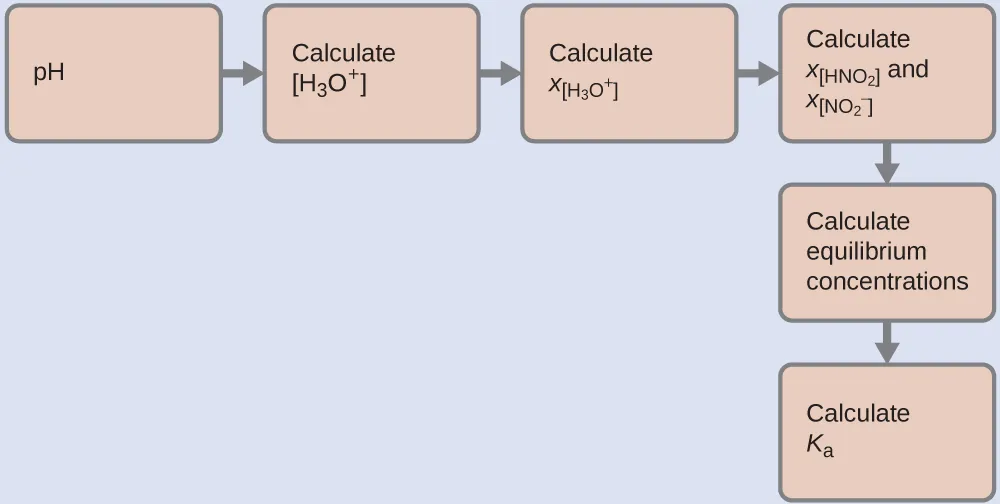To get the various values in the ICE (Initial, Change, Equilibrium) table, we first calculate $[H3O+],[H3O+],$ the equilibrium concentration of $H3O+,H3O+,$ from the pH:

$[H3O+]=10−2.34=0.0046M[H3O+]=10−2.34=0.0046M$
14.74

The change in concentration of $H3O+,H3O+,$ $x[H3O+],x[H3O+],$ is the difference between the equilibrium concentration of H3O+, which we determined from the pH, and the initial concentration, $[H3O+]i.[H3O+]i.$ The initial concentration of $H3O+H3O+$ is its concentration in pure water, which is so much less than the final concentration that we approximate it as zero (~0).

The change in concentration of $NO2−NO2−$ is equal to the change in concentration of $[H3O+].[H3O+].$ For each 1 mol of $H3O+H3O+$ that forms, 1 mol of $NO2−NO2−$ forms. The equilibrium concentration of HNO2 is equal to its initial concentration plus the change in its concentration.

Now we can fill in the ICE table with the concentrations at equilibrium, as shown here: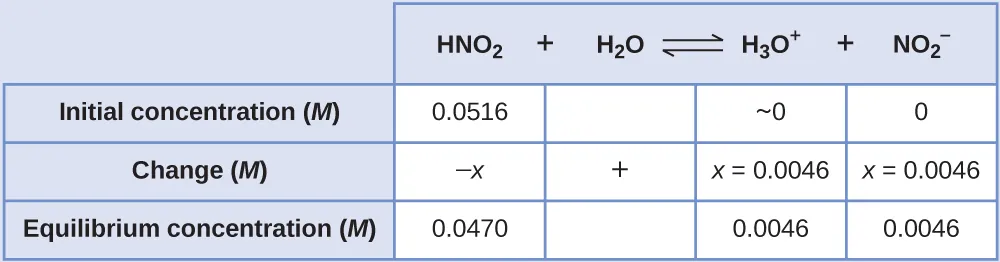Finally, we calculate the value of the equilibrium constant using the data in the table:

$Ka=[H3O+][NO2−][HNO2]=(0.0046)(0.0046)(0.0470)=4.5×10−4Ka=[H3O+][NO2−][HNO2]=(0.0046)(0.0046)(0.0470)=4.5×10−4$
14.75

The pH of a solution of household ammonia, a 0.950-M solution of NH3, is 11.612. What is Kb for NH3.

Kb = 1.8 $××$ 10−5

### Example 14.12

#### Equilibrium Concentrations in a Solution of a Weak Acid

Formic acid, HCO2H, is the irritant that causes the body’s reaction to ant stings (Figure 14.12).
Figure 14.12 The pain of an ant’s sting is caused by formic acid. (credit: John Tann)

What is the concentration of hydronium ion and the pH in a 0.534-M solution of formic acid?

$HCO2H(aq)+H2O(l)⇌H3O+(aq)+HCO2−(aq)Ka=1.8×10−4HCO2H(aq)+H2O(l)⇌H3O+(aq)+HCO2−(aq)Ka=1.8×10−4$
14.76

#### Solution

1. Step 1.
Determine x and equilibrium concentrations. The equilibrium expression is:
$HCO2H(aq)+H2O(l)⇌H3O+(aq)+HCO2−(aq)HCO2H(aq)+H2O(l)⇌H3O+(aq)+HCO2−(aq)$
14.77

The concentration of water does not appear in the expression for the equilibrium constant, so we do not need to consider its change in concentration when setting up the ICE table.
The table shows initial concentrations (concentrations before the acid ionizes), changes in concentration, and equilibrium concentrations follows (the data given in the problem appear in color):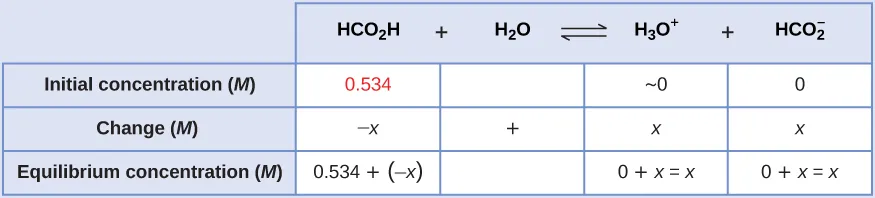2. Step 2.
Solve for x and the equilibrium concentrations. At equilibrium:
$Ka=1.8×10−4=[H3O+][HCO2−][HCO2H]Ka=1.8×10−4=[H3O+][HCO2−][HCO2H]$
14.78
$=(x)(x)0.534−x=1.8×10−4=(x)(x)0.534−x=1.8×10−4$
14.79

Now solve for x. Because the initial concentration of acid is reasonably large and Ka is very small, we assume that x << 0.534, which permits us to simplify the denominator term as (0.534 − x) = 0.534. This gives:

$Ka=1.8×10−4=x20.534Ka=1.8×10−4=x20.534$
14.80

Solve for x as follows:

$x2=0.534×(1.8×10−4)=9.6×10−5x2=0.534×(1.8×10−4)=9.6×10−5$
14.81
$x=9.6×10−5x=9.6×10−5$
14.82
$=9.8×10−3=9.8×10−3$
14.83

To check the assumption that x is small compared to 0.534, we calculate:

$x0.534=9.8×10−30.534=1.8×10−2(1.8%of 0.534)x0.534=9.8×10−30.534=1.8×10−2(1.8%of 0.534)$
14.84

x is less than 5% of the initial concentration; the assumption is valid.

We find the equilibrium concentration of hydronium ion in this formic acid solution from its initial concentration and the change in that concentration as indicated in the last line of the table:

$[H3O+]=~0+x=0+9.8×10−3M.[H3O+]=~0+x=0+9.8×10−3M.$
14.85
$=9.8×10−3M=9.8×10−3M$
14.86

The pH of the solution can be found by taking the negative log of the $[H3O+],[H3O+],$ so:

$−log(9.8×10−3)=2.01−log(9.8×10−3)=2.01$
14.87

Only a small fraction of a weak acid ionizes in aqueous solution. What is the percent ionization of acetic acid in a 0.100-M solution of acetic acid, CH3CO2H?
$CH3CO2H(aq)+H2O(l)⇌H3O+(aq)+CH3CO2−(aq)Ka=1.8×10−5CH3CO2H(aq)+H2O(l)⇌H3O+(aq)+CH3CO2−(aq)Ka=1.8×10−5$
14.88

(Hint: Determine $[CH3CO2−][CH3CO2−]$ at equilibrium.) Recall that the percent ionization is the fraction of acetic acid that is ionized $××$ 100, or $[CH3CO2−][CH3CO2H]initial×100.[CH3CO2−][CH3CO2H]initial×100.$

percent ionization = 1.3%

The following example shows that the concentration of products produced by the ionization of a weak base can be determined by the same series of steps used with a weak acid.

### Example 14.13

#### Equilibrium Concentrations in a Solution of a Weak Base

Find the concentration of hydroxide ion in a 0.25-M solution of trimethylamine, a weak base:
$(CH3)3N(aq)+H2O(l)⇌(CH3)3NH+(aq)+OH−(aq)Kb=6.3×10−5(CH3)3N(aq)+H2O(l)⇌(CH3)3NH+(aq)+OH−(aq)Kb=6.3×10−5$
14.89

#### Solution

This problem requires that we calculate an equilibrium concentration by determining concentration changes as the ionization of a base goes to equilibrium. The solution is approached in the same way as that for the ionization of formic acid in Example 14.12. The reactants and products will be different and the numbers will be different, but the logic will be the same:1. Step 1. Determine x and equilibrium concentrations. The table shows the changes and concentrations: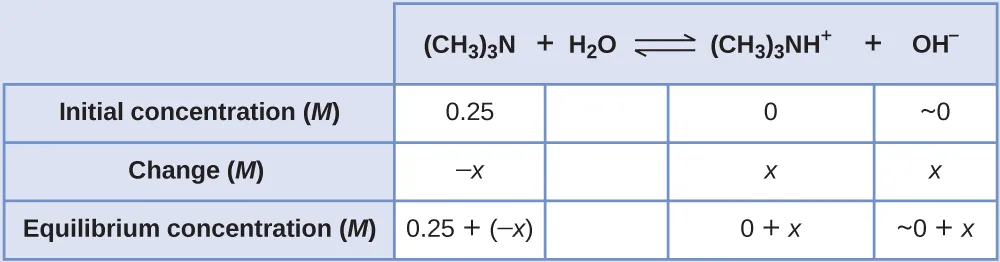2. Step 2.
Solve for x and the equilibrium concentrations. At equilibrium:
$Kb=[(CH3)3NH+][OH−][(CH3)3N]=(x)(x)0.25−x=6.3×10−5Kb=[(CH3)3NH+][OH−][(CH3)3N]=(x)(x)0.25−x=6.3×10−5$
14.90

If we assume that x is small relative to 0.25, then we can replace (0.25 − x) in the preceding equation with 0.25. Solving the simplified equation gives:
$x=4.0×10−3x=4.0×10−3$
14.91

This change is less than 5% of the initial concentration (0.25), so the assumption is justified.
Recall that, for this computation, x is equal to the equilibrium concentration of hydroxide ion in the solution (see earlier tabulation):
$[OH−]=~0+x=x=4.0×10−3M[OH−]=~0+x=x=4.0×10−3M$
14.92

$=4.0×10−3M=4.0×10−3M$
14.93

Then calculate pOH as follows:
$pOH=−log(4.0×10−3)=2.40pOH=−log(4.0×10−3)=2.40$
14.94

Using the relation introduced in the previous section of this chapter:
$pH+pOH=pKw=14.00pH+pOH=pKw=14.00$
14.95

permits the computation of pH:
$pH=14.00−pOH=14.00−2.40=11.60pH=14.00−pOH=14.00−2.40=11.60$
14.96
3. Step 3. Check the work. A check of our arithmetic shows that Kb = 6.3 $××$ 10−5.

(a) Show that the calculation in Step 2 of this example gives an x of 4.0 $××$ 10−3 and the calculation in Step 3 shows Kb = 6.3 $××$ 10−5.

(b) Find the concentration of hydroxide ion in a 0.0325-M solution of ammonia, a weak base with a Kb of 1.76 $××$ 10−5. Calculate the percent ionization of ammonia, the fraction ionized $××$ 100, or $[NH4+][NH3]×100[NH4+][NH3]×100$

7.56 $××$ 10−4 M, 2.33%

Some weak acids and weak bases ionize to such an extent that the simplifying assumption that x is small relative to the initial concentration of the acid or base is inappropriate. As we solve for the equilibrium concentrations in such cases, we will see that we cannot neglect the change in the initial concentration of the acid or base, and we must solve the equilibrium equations by using the quadratic equation.

### Example 14.14

#### Equilibrium Concentrations in a Solution of a Weak Acid

Sodium bisulfate, NaHSO4, is used in some household cleansers because it contains the $HSO4−HSO4−$ ion, a weak acid. What is the pH of a 0.50-M solution of $HSO4−?HSO4−?$
$HSO4−(aq)+H2O(l)⇌H3O+(aq)+SO42−(aq)Ka=1.2×10−2HSO4−(aq)+H2O(l)⇌H3O+(aq)+SO42−(aq)Ka=1.2×10−2$
14.97

#### Solution

We need to determine the equilibrium concentration of the hydronium ion that results from the ionization of $HSO4−HSO4−$ so that we can use $[H3O+][H3O+]$ to determine the pH. As in the previous examples, we can approach the solution by the following steps: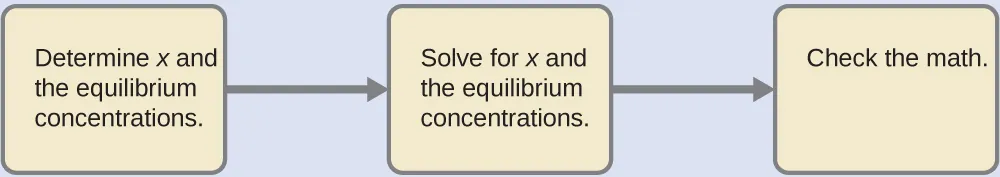1. Step 1. Determine x and equilibrium concentrations. This table shows the changes and concentrations: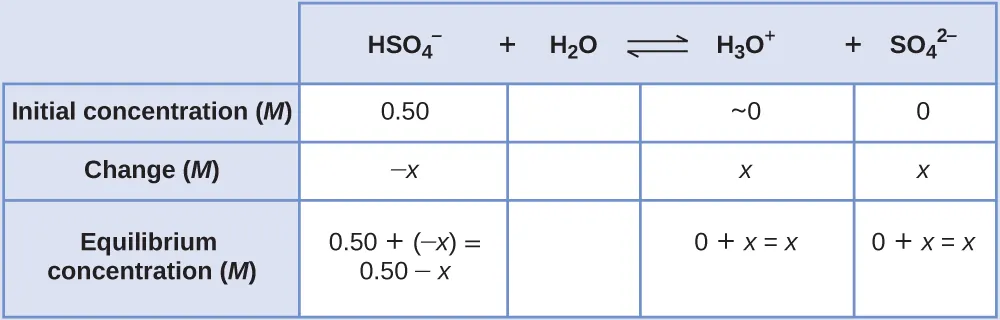2. Step 2.
Solve for x and the concentrations. As we begin solving for x, we will find this is more complicated than in previous examples. As we discuss these complications we should not lose track of the fact that it is still the purpose of this step to determine the value of x.
At equilibrium:
$Ka=1.2×10−2=[H3O+][SO42−][HSO4−]=(x)(x)0.50−xKa=1.2×10−2=[H3O+][SO42−][HSO4−]=(x)(x)0.50−x$
14.98

If we assume that x is small and approximate (0.50 − x) as 0.50, we find:
$x=7.7×10−2x=7.7×10−2$
14.99

When we check the assumption, we calculate:
$x[HSO4−]ix[HSO4−]i$
14.100

$x0.50=7.7×10−20.50=0.15(15%)x0.50=7.7×10−20.50=0.15(15%)$
14.101

The value of x is not less than 5% of 0.50, so the assumption is not valid. We need the quadratic formula to find x.
The equation:
$Ka=1.2×10−2=(x)(x)0.50−xKa=1.2×10−2=(x)(x)0.50−x$
14.102

gives
$6.0×10−3−1.2×10−2x=x2+6.0×10−3−1.2×10−2x=x2+$
14.103

or
$x2++1.2×10−2x−6.0×10−3=0x2++1.2×10−2x−6.0×10−3=0$
14.104

This equation can be solved using the quadratic formula. For an equation of the form
$ax2++bx+c=0,ax2++bx+c=0,$
14.105

x is given by the equation:
$x=−b±b2+−4ac2ax=−b±b2+−4ac2a$
14.106

In this problem, a = 1, b = 1.2 $××$ 10−3, and c = −6.0 $××$ 10−3.
Solving for x gives a negative root (which cannot be correct since concentration cannot be negative) and a positive root:
$x=7.2×10−2x=7.2×10−2$
14.107

Now determine the hydronium ion concentration and the pH:
$[H3O+]=~0+x=0+7.2×10−2M[H3O+]=~0+x=0+7.2×10−2M$
14.108

$=7.2×10−2M=7.2×10−2M$
14.109

The pH of this solution is:
$pH=−log[H3O+]=−log7.2×10−2=1.14pH=−log[H3O+]=−log7.2×10−2=1.14$
14.110

(a) Show that the quadratic formula gives x = 7.2 $××$ 10−2.

(b) Calculate the pH in a 0.010-M solution of caffeine, a weak base:

$C8H10N4O2(aq)+H2O(l)⇌C8H10N4O2H+(aq)+OH−(aq)Kb=2.5×10−4C8H10N4O2(aq)+H2O(l)⇌C8H10N4O2H+(aq)+OH−(aq)Kb=2.5×10−4$
14.111

(Hint: It will be necessary to convert [OH] to $[H3O+][H3O+]$ or pOH to pH toward the end of the calculation.)

pH 11.16

### The Relative Strengths of Strong Acids and Bases

Strong acids, such as HCl, HBr, and HI, all exhibit the same strength in water. The water molecule is such a strong base compared to the conjugate bases Cl, Br, and I that ionization of these strong acids is essentially complete in aqueous solutions. In solvents less basic than water, we find HCl, HBr, and HI differ markedly in their tendency to give up a proton to the solvent. For example, when dissolved in ethanol (a weaker base than water), the extent of ionization increases in the order HCl < HBr < HI, and so HI is demonstrated to be the strongest of these acids. The inability to discern differences in strength among strong acids dissolved in water is known as the leveling effect of water.

Water also exerts a leveling effect on the strengths of strong bases. For example, the oxide ion, O2−, and the amide ion, $NH2−,NH2−,$ are such strong bases that they react completely with water:

$O2−(aq)+H2O(l)⟶OH−(aq)+OH−(aq)O2−(aq)+H2O(l)⟶OH−(aq)+OH−(aq)$
14.112
$NH2−(aq)+H2O(l)⟶NH3(aq)+OH−(aq)NH2−(aq)+H2O(l)⟶NH3(aq)+OH−(aq)$
14.113

Thus, O2− and $NH2−NH2−$ appear to have the same base strength in water; they both give a 100% yield of hydroxide ion.

### Effect of Molecular Structure on Acid-Base Strength

In the absence of any leveling effect, the acid strength of binary compounds of hydrogen with nonmetals (A) increases as the H-A bond strength decreases down a group in the periodic table. For group 17, the order of increasing acidity is HF < HCl < HBr < HI. Likewise, for group 16, the order of increasing acid strength is H2O < H2S < H2Se < H2Te.

Across a row in the periodic table, the acid strength of binary hydrogen compounds increases with increasing electronegativity of the nonmetal atom because the polarity of the H-A bond increases. Thus, the order of increasing acidity (for removal of one proton) across the second row is CH4 < NH3 < H2O < HF; across the third row, it is SiH4 < PH3 < H2S < HCl (see Figure 14.13).

Figure 14.13 As you move from left to right and down the periodic table, the acid strength increases. As you move from right to left and up, the base strength increases.

Compounds containing oxygen and one or more hydroxyl (OH) groups can be acidic, basic, or amphoteric, depending on the position in the periodic table of the central atom E, the atom bonded to the hydroxyl group. Such compounds have the general formula OnE(OH)m, and include sulfuric acid, O2S(OH)2, sulfurous acid, OS(OH)2, nitric acid, O2NOH, perchloric acid, O3ClOH, aluminum hydroxide, Al(OH)3, calcium hydroxide, Ca(OH)2, and potassium hydroxide, KOH: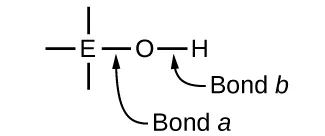If the central atom, E, has a low electronegativity, its attraction for electrons is low. Little tendency exists for the central atom to form a strong covalent bond with the oxygen atom, and bond a between the element and oxygen is more readily broken than bond b between oxygen and hydrogen. Hence bond a is ionic, hydroxide ions are released to the solution, and the material behaves as a base—this is the case with Ca(OH)2 and KOH. Lower electronegativity is characteristic of the more metallic elements; hence, the metallic elements form ionic hydroxides that are by definition basic compounds.

If, on the other hand, the atom E has a relatively high electronegativity, it strongly attracts the electrons it shares with the oxygen atom, making bond a relatively strongly covalent. The oxygen-hydrogen bond, bond b, is thereby weakened because electrons are displaced toward E. Bond b is polar and readily releases hydrogen ions to the solution, so the material behaves as an acid. High electronegativities are characteristic of the more nonmetallic elements. Thus, nonmetallic elements form covalent compounds containing acidic −OH groups that are called oxyacids.

Increasing the oxidation number of the central atom E also increases the acidity of an oxyacid because this increases the attraction of E for the electrons it shares with oxygen and thereby weakens the O-H bond. Sulfuric acid, H2SO4, or O2S(OH)2 (with a sulfur oxidation number of +6), is more acidic than sulfurous acid, H2SO3, or OS(OH)2 (with a sulfur oxidation number of +4). Likewise nitric acid, HNO3, or O2NOH (N oxidation number = +5), is more acidic than nitrous acid, HNO2, or ONOH (N oxidation number = +3). In each of these pairs, the oxidation number of the central atom is larger for the stronger acid (Figure 14.14).

Figure 14.14 As the oxidation number of the central atom E increases, the acidity also increases.

Hydroxy compounds of elements with intermediate electronegativities and relatively high oxidation numbers (for example, elements near the diagonal line separating the metals from the nonmetals in the periodic table) are usually amphoteric. This means that the hydroxy compounds act as acids when they react with strong bases and as bases when they react with strong acids. The amphoterism of aluminum hydroxide, which commonly exists as the hydrate Al(H2O)3(OH)3, is reflected in its solubility in both strong acids and strong bases. In strong bases, the relatively insoluble hydrated aluminum hydroxide, Al(H2O)3(OH)3, is converted into the soluble ion, $[Al(H2O)2(OH)4]−,[Al(H2O)2(OH)4]−,$ by reaction with hydroxide ion:

$Al(H2O)3(OH)3(aq)+OH−(aq)⇌H2O(l)+[Al(H2O)2(OH)4]−(aq)Al(H2O)3(OH)3(aq)+OH−(aq)⇌H2O(l)+[Al(H2O)2(OH)4]−(aq)$
14.114

In this reaction, a proton is transferred from one of the aluminum-bound H2O molecules to a hydroxide ion in solution. The Al(H2O)3(OH)3 compound thus acts as an acid under these conditions. On the other hand, when dissolved in strong acids, it is converted to the soluble ion $[Al(H2O)6]3+[Al(H2O)6]3+$ by reaction with hydronium ion:

$3H3O+(aq)+Al(H2O)3(OH)3(aq)⇌Al(H2O)63+(aq)+3H2O(l)3H3O+(aq)+Al(H2O)3(OH)3(aq)⇌Al(H2O)63+(aq)+3H2O(l)$
14.115

In this case, protons are transferred from hydronium ions in solution to Al(H2O)3(OH)3, and the compound functions as a base.

Order a print copy

As an Amazon Associate we earn from qualifying purchases.